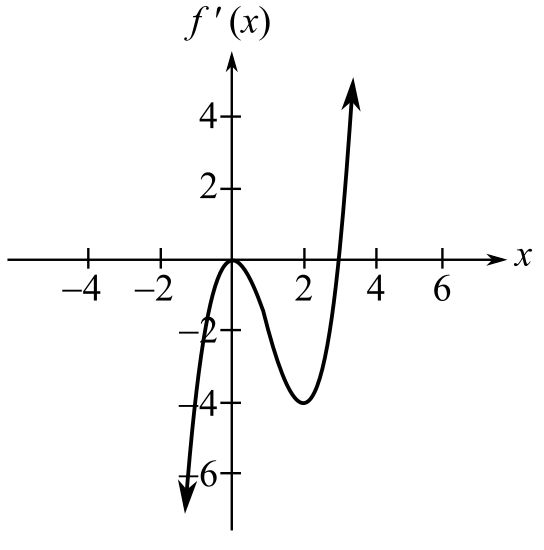Chapter 10.1, Problem 45E### Mathematical Applications for the ...

11th Edition
Ronald J. Harshbarger + 1 other
ISBN: 9781305108042

#### Solutions

Chapter
Section### Mathematical Applications for the ...

11th Edition
Ronald J. Harshbarger + 1 other
ISBN: 9781305108042
Textbook Problem

# In each of Problems 43-46, a graph of f ' ( x ) is given. Use the graph to determine the critical values of f ( x ) , where f ( x ) is increasing, where it is decreasing, and where it has relative maxima, relative minima, and horizontal points of inflection. In each case, sketch a possible graph for f ( x ) that passes through (0, 0). f ' ( x ) = x 3 − 3 x 2To determine

The intervals of x-values where the function derivative y=x33x2 is increasing and where it is decreasing, the coordinates of the relative maxima, relative minima, or horizontal points of inflection from the following graph:Explanation

Given Information:

The provided equation is y=x33x2.

Explanation:

The critical values are the only values at which the graph can have turning points, the derivative cannot change sign anywhere except at the critical value.

This, in an interval between two critical values, the sign of the derivative at any value in the interval will be the sign of the derivative at all values in the interval.

As per the First Derivative Test,

The first derivative of the function is evaluated. The first derivative is made equal to zero in order to get the critical points.

The values of the critical values are kept inside the original function which gives the critical points. The intervals of the values of x are then evaluated for the relative maximum and minimum.

Consider the provided equation y=x33x2,

The critical values are the only values at which the graph can have turning points, the derivative cannot change sign anywhere except at the critical value.

Hence, there will no change in the values of critical values as in the derivative graph.

Put the value of y=0,

y=x33x2=0

Evaluate the values of x from the equation:

x33x2=0x2(x3)=0

Hence, the value of x is x=0,3.

Test the derivative at the left and right sides of all the critical points.

Left of 0 is x=1, On the right is x=1.

Left of 3 is x=1, On the right is x=4.

Check these points by putting in the first derivative.

At x=1,

y=(1)33(1)2=13=4

Here, f(x)<0.

At x=1,

y=(1)33(1)2=13=2

Here, f(x)<0.

At x=4,

y=(4)33(4)2=6448=16

Here, f(x)>0

### Still sussing out bartleby?

Check out a sample textbook solution.

See a sample solution

#### The Solution to Your Study Problems

Bartleby provides explanations to thousands of textbook problems written by our experts, many with advanced degrees!

Get Started

#### Sketch the graphs of the equations in Exercises 512. xy=x2+1

Finite Mathematics and Applied Calculus (MindTap Course List)

#### find the real roots of each equation by factoring. 132. 6x2 + x + 12 = 0

Applied Calculus for the Managerial, Life, and Social Sciences: A Brief Approach

#### If n(A)=4, n(B)=5 and n(AB)=9, find n(AB).

Finite Mathematics for the Managerial, Life, and Social Sciences

#### The polar form for the graph at the right is:

Study Guide for Stewart's Single Variable Calculus: Early Transcendentals, 8th